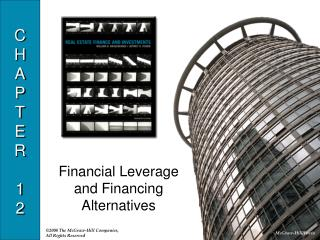Download PresentationCHAPTER 12

# CHAPTER 12 - PowerPoint PPT PresentationDownload Presentation## CHAPTER 12

- - - - - - - - - - - - - - - - - - - - - - - - - - - E N D - - - - - - - - - - - - - - - - - - - - - - - - - - -
##### Presentation Transcript

1. CHAPTER12 Financial Leverage and Financing Alternatives

2. Financial Leverage • What is financial leverage? • Benefit of borrowing at a lower interest rate than the rate of return on the property. • Why use financial leverage? • Diversification benefits of lower equity investment • Can invest in other property • Mortgage interest tax benefit • Magnify returns if the return on the property exceeds the cost of debt

3. Financial Leverage: Before-Tax • Positive Financial Leverage • Returns are higher with debt • Unlevered BTIRR • Return with no debt • If unlevered BTIRR > interest rate on debt • The BTIRR on equity increases with debt. • There is positive financial leverage.

4. Financial Leverage: Before-Tax • Equation 1: • BTIRRE= BTIRRP + (BTIRRP – BTIRRD)(D/E) • BTIRRE = Before-Tax IRR on equity invested • BTIRRP = Before-Tax IRR on total investment in the property • BTIRRD = Before-Tax IRR on debt (effective cost including points) • D/E =Debt/Equity ratio

5. Financial Leverage: Before-Tax • Equation 1 shows that as long as: • BTIRRP > BTIRRD, thenBTIRRE > BTIRRP • This implies increasing D/E…… • But the use of debt is limited • Debt coverage ratio restrictions • Higher loan to value ratios are riskier to lenders…leading to higher interest rates • Higher debt levels increase risk to equity investor

6. Financial Leverage: Before-Tax • Negative Financial Leverage • If BTIRRD > BTIRRP, thenBTIRRE < BTIRRP • The use of debt reduces the return on equity.

7. Financial Leverage: After-Tax • Equation 2: • ATIRRE= ATIRRP + (ATIRRP – ATIRRD)(D/E) • ATIRRE = After-Tax IRR on equity invested • ATIRRP = After-Tax IRR on total investment in the property • ATIRRD = BTIRRD (1-t) • After-Tax IRR on debt (effective cost after taxes including points) • D/E =Debt/Equity

8. Financial Leverage Example • Building Value=\$85,000, Land Value=\$15,000, Total Value=\$100,000 • Loan Assumptions: • Loan Amount=\$80,000 • Interest Rate=10% • Term: Interest Only • 80% LTV • Income Assumptions: • NOI=\$12,000 per year (level) • Income tax rate=28% • Depreciation=31.5 years (straight line) • Resale price= \$100,000 • Holding period=5 years

9. Cash Flow Summary and IRR With Leverage

10. Cash Flow Summary and IRR Without Leverage

11. Financial Leverage: After-Tax • Break-even interest rate • Maximum interest rate before negative financial leverage • ATIRRD= ATIRRP • ATIRRD= BTIRRD(1-t) • BTIRRD= = • Risk considerations

12. Break-even Interest Rate

13. Alternative Financing Structures • Sale-Leaseback of Land and Ground Leases • Own building and lease land from a different investor • Motivations • 100% financing possible • Lease payments are tax deductible • Building is depreciable; land is not • Possible purchase option at end of lease